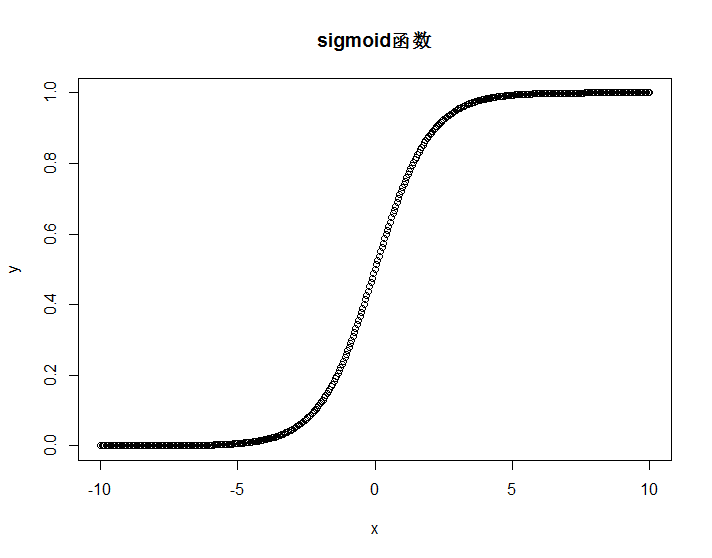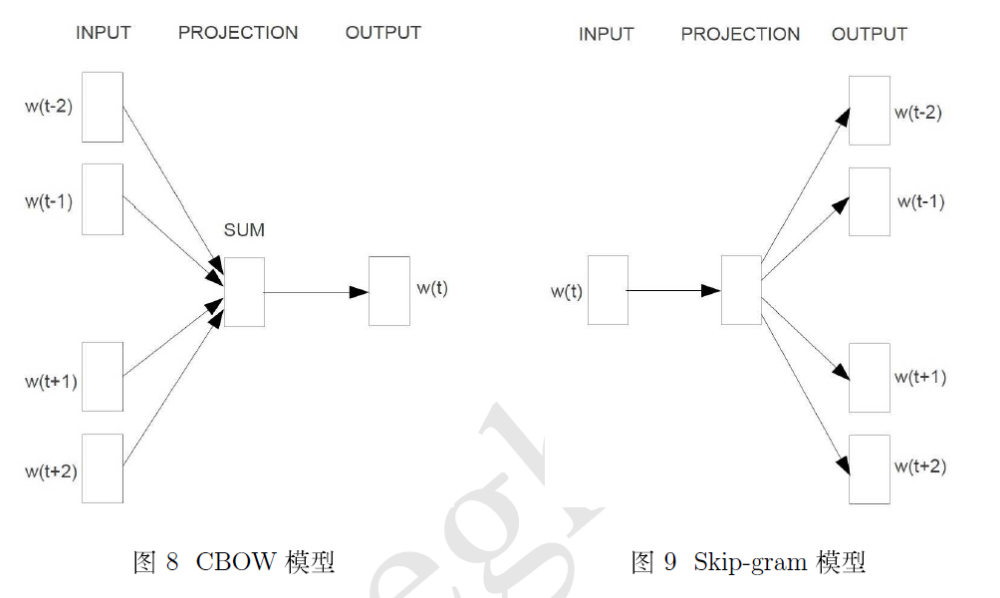@evilking 2018-04-30T16:55:15.000000Z 字数 6265 阅读 419

NLP

# Word2Vec之 预备知识

## 预备知识

### Sigmoid函数

Sigmoid函数是神经网络中常用的激活函数之一，定义为

x <- seq(-10,10,0.05)y <- 1/(1 + exp(-x))plot(x,y,'p',main = "sigmoid函数",)Sigmoid函数的导函数具有如下形式：

$\sigma'(x) = \left( \frac{1}{1 + e^{-x}} \right)' = \left( -1 \times \frac{1}{(1 + e^{-x})^2} \right) \left( 1 + e^{-x} \right)' \\\ \ \ \ = \left( -1 \times \frac{1}{(1 + e^{-x})^2} \right) \times e^{-x}(-1) \\\ \ \ \ = \frac{1}{1 + e^{-x}} \times \frac{e^{-x}}{1 + e^{-x}} \\\ \ \ \ = \sigma(x) \times (1 - \sigma(x)$

### Huffman树

Huffman树是由Huffman编码生成；核心思想就是让出现频率较高的元素具有较短的编码，出现频率较低的元素具有较长编码，这样整体集合的编码长度就比较短了，因为集合中大部分都是出现频率较高的元素.

#### 树的基本概念

• 路径和路径长度
在一棵树中，从一个结点往下可以达到的孩子或孙子结点之间的通路，称为路径.通路中分支的数目称为路径长度.
若规定根结点的层号为 1，则从根结点到第 $L$ 层结点的路径长度为 $L - 1$

• 结点的权和带权路径长度
若为树中结点赋予一个具有某种含义的(非负)数值，则这个数值称为该结点的权.
结点的带权路径长度是指，从根结点到该结点之间的路径长度与该结点的权的乘积.

• 树的带权路径长度
树的带权路径长度规定为所有叶子结点的带权路径长度之和.

#### Huffman树的构造

1. $\{\omega_1,\omega_2,\cdots,\omega_n\}$ 看成是有 $n$ 棵树的森林（每棵树仅有一个结点）

2. 在森林中选出两个根节点的权值最小的树合并，作为一棵新树的左、右子树，且新树的根结点权值为其左、右子树根结点权值之和

3. 从森林中删除选取的两棵树，并将新树加入森林

4. 重复 2, 3 步，直到森林中只剩一棵树为止，该树即为所求的 Huffman 树

## 自然语言背景知识

word2vec 是用来生成词向量的工具，而词向量与语言模型有着密切的关系，所以我们先介绍一下语言模型和词向量的概念.

### n-gram 模型

$n = 2$ 为例，就有

### 词向量

word2vec 中就是采用的 Distributed Representation 词向量方式，具体如何操作，在后面会介绍.

## word2vec模型介绍

word2vec 中有两个重要的模型——CBOW(Continuous Bag-of-Words Model) 模型Skip-gram(Continuous Skip-gram Model) 模型.• 私有
• 公开
• 删除